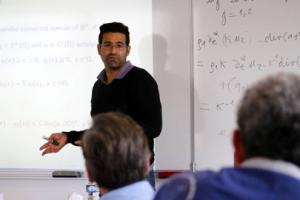Determination of Time-Dependent Coefficients for Hyperbolic Equations
Speaker
A/Prof. Yavar Kian
University of Aix-Marseille, France
Abstract

Let Ω be a C2  bounded domain of Rn , n ≥ 2, and fix Q = (0, T ) × Ω with T > 0. We consider the inverse problem of determining a time-dependent damping coefficient a and a time- dependent potential q, appearing in a Dirichlet initial-boundary value problem for the damped wave equation ∂_t^2u − xu + a(t, x)t u + q(t, x)u = 0 in Q from observations on ∂Q.  Using an approach related to the strategy set for partial  data Calderón problems and based on Carleman estimates, our goal is to reduce as much as possible the information  on ∂Q that  allows to determine general time-dependent coefficients. We consider both results of uniqueness and stability for this problem.